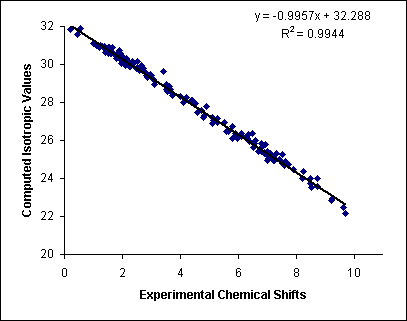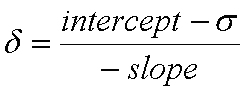# General Information

Computed nuclear magnetic resonance chemical shift values are of great utility in modern chemical research today. Improvements in methods, techniques, and computer hardware are continually increasing the accuracy of computed chemical shifts and are expanding the number and types of chemical systems and problems to which they may be applied. Furthermore, these improvements also serve to make such calculations more accessible to researchers who are non-experts in computational chemistry.

Empirical scaling is one of the many techniques available for achieving high-accuracy computed chemical shifts with low to moderate demand for computational resources and expertise. In this approach, systematic error is decreased by way of scaling factors determined via linear regression analyses of experimental and computed chemical shift data. Generating these scaling factors can require a fair amount of knowledge and computational resources, but once they are determined and made available to researchers, they are relatively simple to utilize and can provide outstanding results.

An example of such a linear regression analysis is shown below. Here computed isotropic shielding constants are plotted against known experimental 1H chemical shifts for a large set of small organic molecules. It is evident that there is a strong linear correlation between the theoretical shielding constants and experimental chemical shifts.The equation of this line provides two useful parameters. The first is the slope of the line, which is an indication of the amount of systematic error in the computational method (a slope of exactly -1 would indicate zero systematic error). The value of the slope can therefore be used to "scale" the results in such a way that systematic error is generally removed. The second parameter is the intercept, which provides an alternative to calculating the shielding constants for a specific reference compound (e.g., TMS), which is the traditional approach in NMR calculations. Computing chemical shifts by direct comparison to TMS has many drawbacks, but the linear regression method provides a reference value that is not only scaled to remove systematic error, but also avoids specific error associated with utilizing a single reference compound.

Once a linear regression analysis has been conducted for a given computational method, it is exceptionally easy to utilize the parameters to achieve high-accuracy chemical shifts from the computed isotropic shielding constants for your molecule of interest. The equation below (which is typically utilized in a spreadsheet application) produces scaled chemical shift values (δ relative to TMS) from the computed isotropic shielding constants (σ) and the slope and intercept from the linear regression analysis.Please see our instructions page for specific details on utilizing scaling factors and our examples page for some examples of how accurate scaled chemical shifts can be.